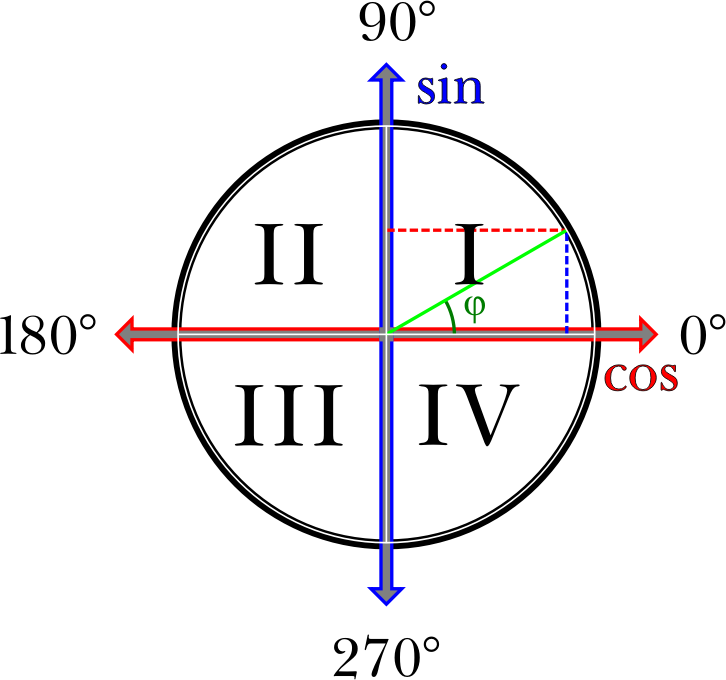## Trigonometry Mnemonic

This image is pretty much a summary of the entire field of trigonometry by illustrating all of its principles. At least that was my goal when I drew it.

Obviously, not everything we know of trigonometry can be explicitly present in a single image. But referring back to this image helps a student understand even things not explicitly mentioned in it, e.g. tangents, secants, arc length, area of the arc cutout, etc.

It presents the unit circle drawn over the planar Cartesian coordinates, with their division of the plane into four quadrants denoted by the Roman numerals I-IV. It shows the four principle angles in degrees, starting with the 0° angle at the rightmost point on the circle, growing as we move around the circle in the counterclockwise direction, eventually coming back to where it started and becoming 0° again because the degrees are presented modulo 360 (so 360° is the same as 0°).

It shows that the X-axis represents the cosine (cos) of the angle, while the Y-coordinate represents the sine (sin) of the angle.

That makes it easy to remember that the sine is positive when the Y-coordinate is positive, which is in Qudrants I and II. The sine is negative, when the Y-coordinate is negative, i.e., in Quadrants III and IV.

And the cosine is positive when the X-coordinate is positive, in Quadrants I and IV, and negative when the Y-coordinate is negative, in Quadrants II and III.

It illustrates that the square of the sine added to the square of the cosine is always 1. That is because the radius of the unit circle is 1, which makes the hypotenuse of the right-angle triangle formed by the radius and the X- and Y-coordinates. Therefore, based on the Pythagorean theorem the square of the sine added to the square of the cosine is always 1.

That also means the sine and the cosine do not derive from the angle in a linear manner.

For example, as drawn φ = 30°, which makes it one third the way from 0° to 90°. But the Y-coordinate at the point the green radius touches the circle is halfway between 0 and 1, so 0.5 and not 0.333... as may or may not seem it should be. Therefore sin φ = 0.5.

If we apply the Pythagorean theorem we can tell that cos φ = square root of (1 - 0.5²), or sqrt(1-0.25) = sqrt(0.75) = sqrt(3/4) = sqrt(3)/2.

And since our φ is in Quadrant I, both the sine and cosine are positive numbers.

There is a lot more to trigonometry and I could write a book about how it is all clear from this one image. But that would not fit into this description, so I shall stop here.

Tags
Filesize
21 k
Safe for Work?
Yes